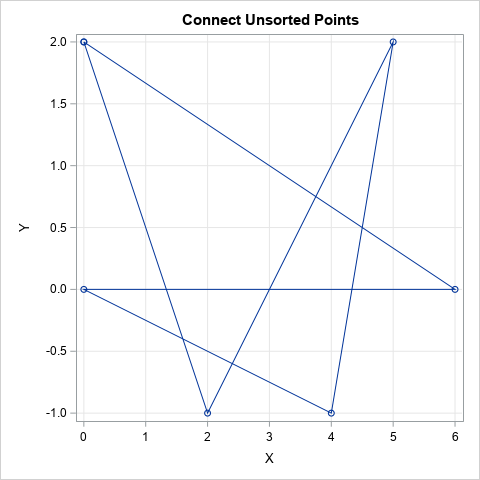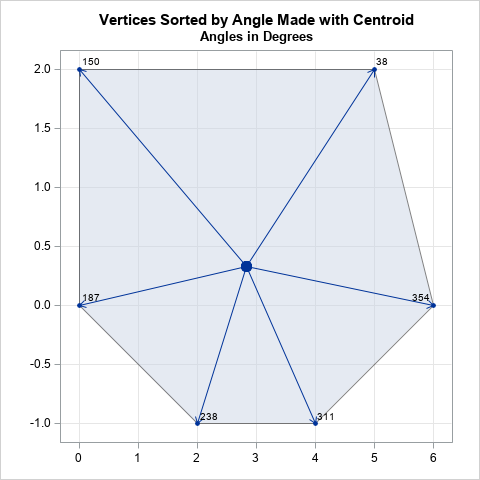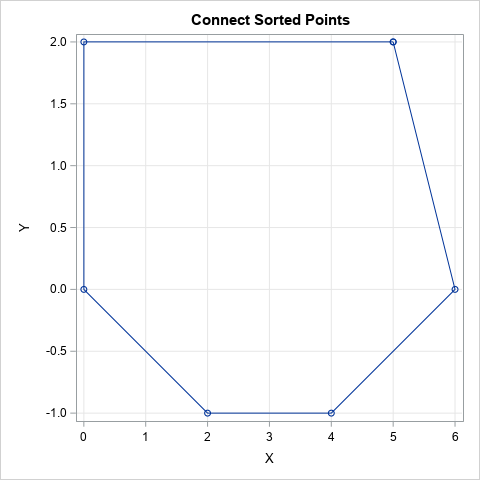In a previous article, I showed how to use the CVEXHULL function in SAS/IML to compute the convex hull of a finite set of planar points. The convex hull is a convex polygon, which is defined by its vertices. To visualize the polygon, you need to know the vertices in sequential order. Fortunately, the CVEXHULL function returns the vertices in counterclockwise order, so you can connect the points in the order they are provided.

But what if the CVEXHULL function did not output the vertices in sequential order? It turns out that you can perform a simple computation that orders the vertices of a convex polygon. This article shows how.

### Connect unsorted vertices of a convex polygon

Let's start by defining six points that form the vertices of a convex polygon. The vertices are not sorted in any way, so if you connect the points in the order given, you get a star-like pattern:

```proc iml; P = { 0 2, 6 0, 0 0, 4 -1, 5 2, 2 -1 };   /* create a helper function to connect vertices in the order they are given */ start GraphVertices(P); Poly = P // P[1,]; /* repeat first point to close the polygon */ call series(Poly[,1], Poly[,2]) procopt="aspect=1" option="markers" grid={x y}; finish; title "Connect Unsorted Points"; run GraphVertices(P);```The line plot does not look like a convex polygon because the vertices were not ordered. However, it is not hard to order the vertices of a convex polygon.

### Order vertices of a convex polygonThe key idea is to recall that the centroid of a polygon is the arithmetic average of the coordinates of its vertices. For a convex polygon, the centroid always lies in the interior of the polygon, as shown in the graph to the right. The centroid is displayed as a large dot in the center of the polygon.

You can use the centroid as the origin and construct the vectors from the centroid to each vertex. For each vector, you can compute the angle made with the horizontal axis. You can then sort the angles, which provides a sequential ordering of the vertices of the convex polygon. In the graph at the right, the angles are given in degrees, but you can use radians for all the calculations. For this example, the angles that the vectors make with the positive X axis are 38 degrees, 150 degrees, 187 degrees, and so forth.

The SAS/IML language provides a compact way to compute the centroid and the vectors. You can use the ATAN2 function in SAS to compute the angles, as follows:

```C = mean(P); /* centroid of a convex polygon */ v = P - C; /* vectors from centroid to points on convex hull */ radian = atan2( v[,2], v[,1] ); /* angle with X axis for each vector */```

At this point, you could sort the vertices by their radian measure. However, the ATAN2 function returns values in the interval (-π π]. If you prefer to order the vertices by using the standard "polar angle," which is in the interval [0, 2π), you can add 2π to any negative angle from the ATAN2 function. You can then use the angles to sort the vertices, as follows:

```/* Optional: The ATAN2 function returns values in (-pi, pi]. Convert to values in (0,2*pi] */ pi = constant('pi'); idx = loc( radian<0 ); radian[idx] = radian[idx] + 2*pi;   /* now sort the points by angle */ call sortndx(idx, radian); /* get row numbers that sort the angles */ Q = P[idx,]; /* sort the vertices of the polygon by their angle */ title "Connect Sorted Points"; run GraphVertices(Q);```With this ordering, the vertices are now sorted in sequential order according to the angle each vertex makes with the centroid.

In summary, the CVEXHULL function in SAS/IML returns vertices of a convex polygon in sequential order. But if you are even given the vertices in a random order, you can perform a computation to sort them by using the angle each vertex makes with the centroid.

The post The order of vertices on a convex polygon appeared first on The DO Loop.